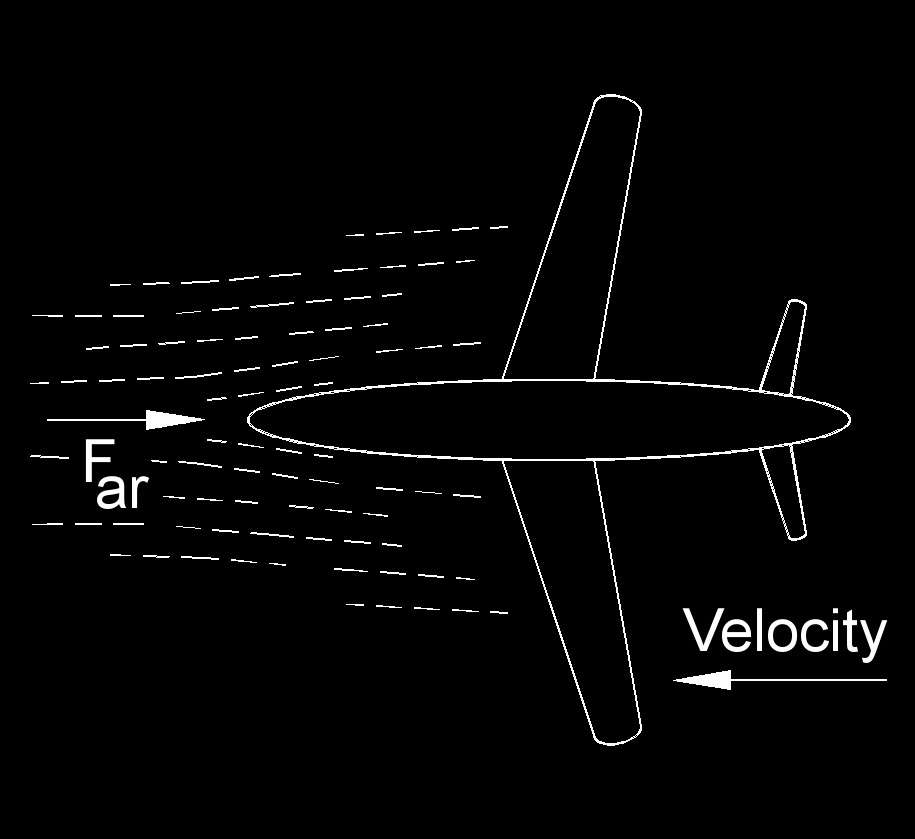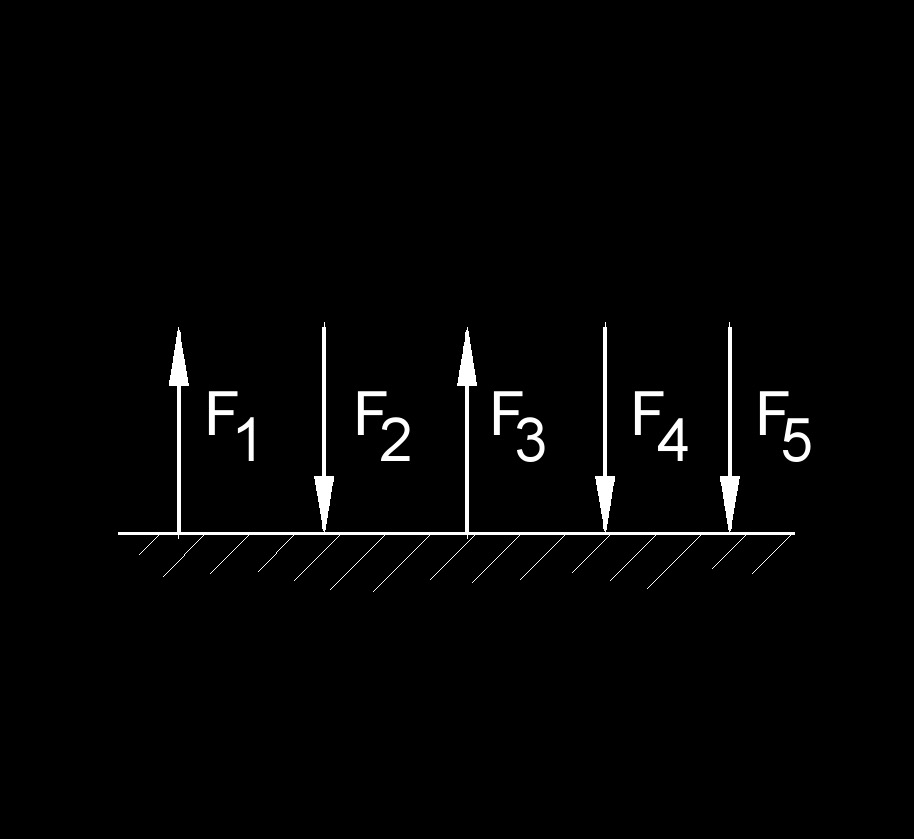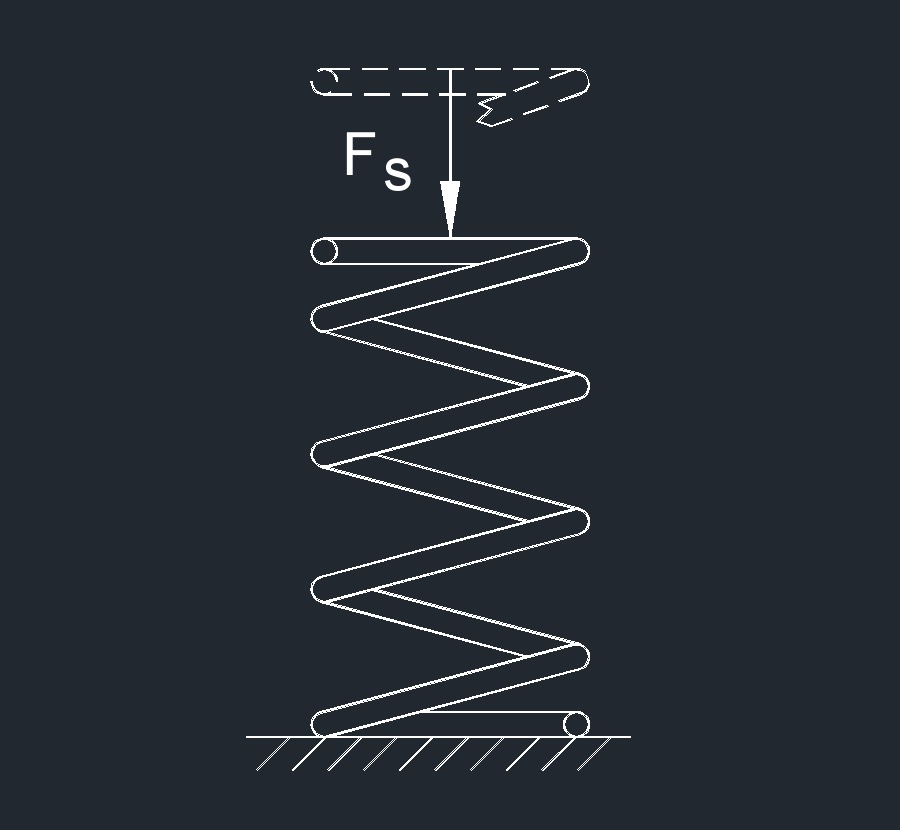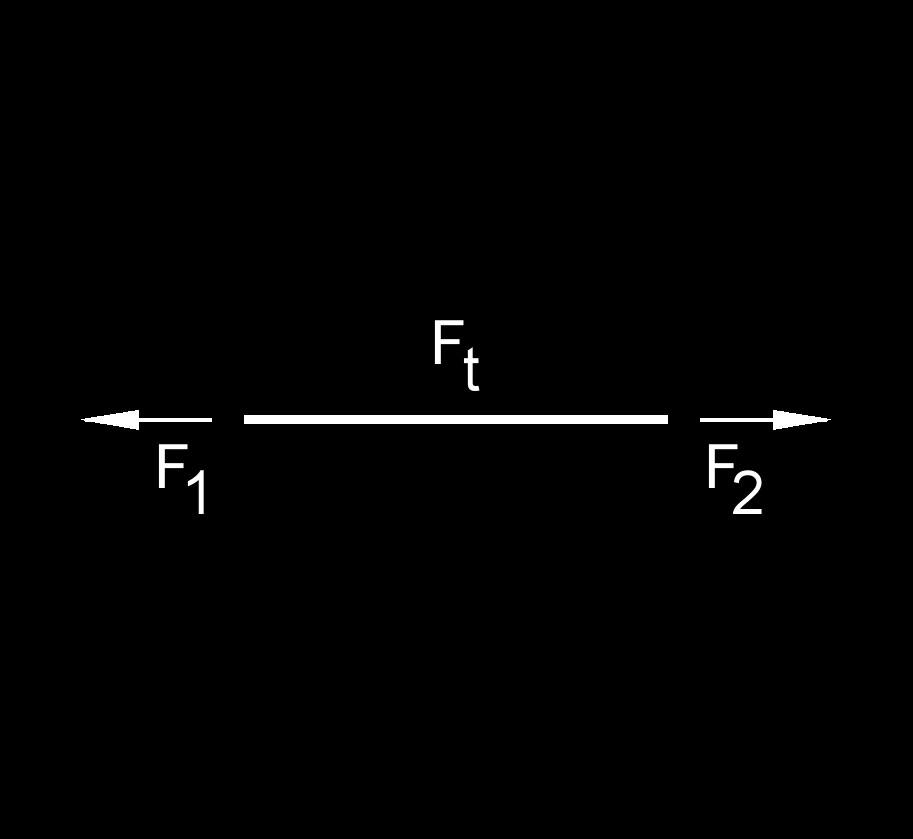# Force

Written by Jerry Ratzlaff on . Posted in Classical MechanicsForce, abbreviated as F, is the push or pull of an object resulting in a change from rest or motion.  So when you apply force to an object the velocity changes, the change in velocity is acceleration.  Force is a vector quantity having magnitude and direction, some of these include acceleration, displacement, drag, lift, momentum, thrust, torque, velocity, and weight.

 (Eq. 1)  $$\large{ F = m \; a }$$ (Eq. 2)  $$\large{ F = p \; A }$$         (Eq. 3)  $$\large{ F = \frac{P}{v} }$$ (Eq. 4)  $$\large{ F = \frac{P \; t}{d} }$$ Where: $$\large{ F }$$ = force $$\large{ a }$$ = acceleration $$\large{ A }$$ = area $$\large{ d }$$ = displacement $$\large{ m }$$ = mass $$\large{ P }$$ = power $$\large{ p }$$ = pressure $$\large{ t }$$ = time $$\large{ v }$$ = velocity

Solve for:

$$\large{ d = \frac {P \; t}{F} }$$

(Eq. 1)  $$\large{ P = F \; v }$$

(Eq. 2)  $$\large{ P = \frac{F \; d}{t} }$$

$$\large{ t = \frac{F \; d}{P} }$$

$$\large{ v = \frac{P}{F} }$$

## General Three-dimensional Force

All other combinations of nonconcurrent, nonparallel and noncoplanar forces.

## Force Types

All forces can be divided into two basic types of forces:

• Contact force
• Air resistance force
• Applied force
• Friction force
• Normal force
• Spring force
• Tension force
• Non-contact force
• Gravity force
• Electric force
• Magnetic force

### Air Resistance ForceAir resistance force, abbreviated as $$F_{ar}$$, is the force in opposition to a moving object through the air.

### Applied ForceApplied force, abbreviated as $$F_a$$, can come from different types of forces, one of them could be Newton's Second Law.  There really is no one formula.

### Centrifugal forceCentrifugal force is when a force pushes away from the center of a circle, but this does not really exist.  When an object travels in a circle, the object always wants to go straight, but the centripetal force keeps the object traveling along an axis of rotation.

### Centripetal forceThe direction of the centripetal force is always toward the center of the circle and perpendicular to the velocity.  Any combination of forces causing a uniform circular motion can be called centripetal force.

### Collinear ForceCollinear forces all share the same line of action.

### Concurrent ForceConcurrent forces are all acting at the same point.

### Coplannar Parallel ForceCoplannar parallel forces can be in the same or opposite direction and are on the same plane.

### Electric ForceAn electric force, abbreviated as $$F_e$$, is an attraction or repulsion force between any two charged objects.

### Friction ForceFriction force, abbreviated as $$F_f$$, is the force an object exerts on the surface of an object as it slides across.

### Gravity ForceGravity force is the force exerted between two masses.

### Magnetic ForceA magnetic force, abbreviated as $$F_m$$, is an attraction or repulsion force between electrically charged particles moving in a magnetic field.

### Non Coplannar ForceAll non coplannar forces lines of action lie on different planes.

### Non Coplannar Concurrent ForceAll non coplannar concurrent forces act at the same point but their lines of action lie on different planes.

### Non Coplannar Non Concurrent ForceAll non coplannar non concurrent forces do not meet and their lines do not lie on the same plane.

### Normal ForceNormal force, abbreviated as $$F_n$$, is the supported force between two contacting objects.

### Spring ForceSpring force, abbreviated as $$F_s$$, is the force that compresses (a repulsive force) or stretches (an attractive force) the spring.

### Tension ForceTension force, abbreviated as $$F_t$$, is the force that is exerted through a rope, string or wire when pulled from opposite directions.

Tags: Equations for Force